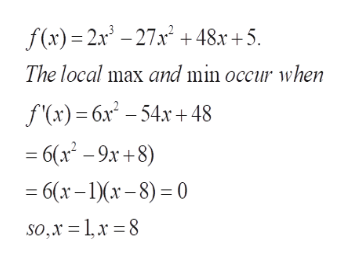The function f(x)=2x3−27x2+48x+5 as one local minimum and one local maximum. Use a graph of the function to estimate these local extrema.This function has a local minimum at x =  with output value=   and a local maximum at x =  with output value=

Question

The function f(x)=2x3−27x2+48x+5 as one local minimum and one local maximum.
Use a graph of the function to estimate these local extrema.

This function has a local minimum at x =

with output value=

and a local maximum at x =

with output value=

Step 1

To estimate (approximately) the local minimum and the local maximum of the given function by graphing

Step 2

The point of the problem is that we need not evaluate the exact values; enough to estimate using the graph.  we know that the extr...help_outlineImage Transcriptionclosef(x)2x3-27x 48x+5 The local max and min occur wvhen S(к) — бх* - 54х +48 6(x-9x+8) — 6(х - 1Xx - 8) -0 so,x = 1,x 8 fullscreen

Want to see the full answer?

See Solution

Want to see this answer and more?

Our solutions are written by experts, many with advanced degrees, and available 24/7

See Solution
Tagged in

Calculus